# Species Diversity¶

EcoPy contains several methods for estimating species diversity:

diversity(x, method='shannon', breakNA=True)

Calculate species diversity for every site in a site x species matrix

Parameters

x: numpy.ndarray or pandas.DataFrame (required)
A site x species matrix, where sites are rows and columns are species.
method: [‘shannon’ | ‘simpson’ | ‘invSimpson’ | ‘dominance’ | ‘spRich’ | ‘even’]

shannon: Calculates Shannon’s H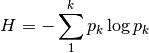where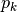is the relative abundance of species k

simpson: Calculates Simpson’s D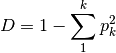invSimpson: Inverse of Simpson’s D

dominance: Dominance index.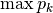spRich: Species richness (# of non-zero columns)

even: Evenness of a site. Shannon’s H divided by log of species richness.

breakNA: [True | False]
Whether null values should halt the process. If False, then null values are removed from all calculations.

Example

Calculate Shannon diversity of the ‘varespec’ dataset from R:

import ecopy as ep
shannonH = ep.diversity(varespec, 'shannon')

rarefy(x, method='rarefy', size=None, breakNA=True)

Returns either rarefied species richness or draws a rarefaction curve for each row. Rarefied species richness is calculated based on the smallest sample (default) or allows user-specified sample sizes.

Parameters

x: numpy.ndarray or pandas.DataFrame (required)
A site x species matrix, where sites are rows and columns are species.
method: [‘rarefy’ | ‘rarecurve’]

rarefy: Returns rarefied species richness.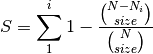where N is the total number of individuals in the site,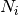is the number of individuals of species i, and size is the sample size for rarefaction

rarecurve: Plots a rarefaction curve for each site (row). The curve is calculated as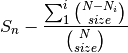where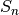is the total number of species in the matrix and size ranges from 0 to the total number of individuals in each site.

Example

Calculate rarefied species richness for the BCI dataset:

import ecopy as ep

ep.rarefy(varespec, 'rarecurve')